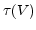GVD_Gate
Subsections

## GVD_Gate

Vh (Volt) :
Inflection point of the sigmoidal function p(V)
Vc (Volt) :
Determines the slope of the sigmoidal function p(V)
Ts :
Scale of the time constantTe :
Additional exponential factor of the time constantnummethod (flag) :
Numerical method for the solution of the differential equation: Exp. Euler = 0, Crank-Nicolson = 1
k (1) :
The exponent of the gate
p :
The state variable.